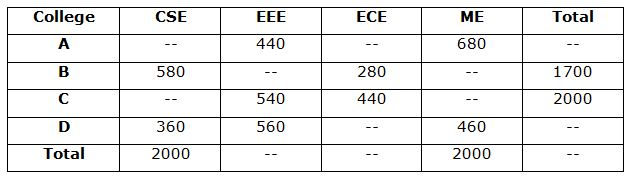# IBPS / LIC Clerk Prelims Quantitative Aptitude Questions 2019 (Day-02)

Dear Aspirants, Our IBPS Guide team is providing new series of Quantitative Aptitude Questions for IBPS Clerk Prelims 2019 so the aspirants can practice it on a daily basis. These questions are framed by our skilled experts after understanding your needs thoroughly. Aspirants can practice these new series questions daily to familiarize with the exact exam pattern and make your preparation effective.

Check here for IBPS Clerk Prelims Mock Test 2019

##### Check here for IBPS Clerk  Mock Test 2019
[WpProQuiz 7211]

Data Interpretation

Directions (1 – 6): Study the following information carefully and answer the questions given below.

The given table shows the number of students in four different colleges in four different departments.1) If the ratio of the number of students of CSE from A to C is 21:32, find the number of ME students from C?

a) 380

b) 360

c) 340

d) 320

e) None of these

2) Total number of EEE students from all the colleges together is 1900, then what is the number of ME students from B?

a) 420

b) 460

c) 440

d) 480

e) None of these

3) If the ratio of the number of ME students from B to C is 24: 19, what is the number of CSE students from C?

a) 580

b) 600

c) 620

d) 660

e) None of these

4) If the total number of students from A is equal to the total number of ME students from all the colleges together. The number of ECE students from C is approximately what percent of the total number of students from A all the departments together?

a) 18%

b) 20%

c) 22%

d) 24%

e) None of these

5) If the average number of ECE students from all the colleges together is 430 and the number of ECE students from D is equal to the number of ME students from A. What is the ratio of the number of ECE students from A to the number of CSE students from D?

a) 7: 8

b) 8: 9

c) 5: 6

d) 4: 3

e) None of these

6) What is the difference between the number of EEE and ME students from B together and the number of CSE and ME students from C together?

a) 180

b) 200

c) 220

d) 140

e) 160

Missing number series

Directions (7 – 10): What value should come in the place of (?) in the following number series?

7) 4, 6, 18, 66, 284, ?

a) 360

b) 972

c) 1280

d) 1450

e) 1620

8) 12, 22, 38, ?, 101.5, 160.25

a) 63

b) 65

c) 67

d) 69

e) 71

9) 18, 54, 79, 95, 104, ?

a) 110

b) 106

c) 108

d) 112

e) 114

10) 995, 723, 505, 335, 207, ?

a) 100

b) 105

c) 110

d) 115

e) 120

Directions (1-6) :

Total number of CSE students =2000

Number of CSE students from A and C = 2000 – 580 – 360 = 1060

Number of CSE students from C = 32/53 * 1060 = 640

Number of ME students from C = 2000 – 640 – 540 – 440 = 380

Number of EEE students from B = 1900 – 440 – 540 – 560 = 360

Number of ME students from B = 1700 – 580 – 360 – 280 = 480

Number of ME students from B and C = 2000 – 680 – 460 = 860

Number of ME students from C = 19/43 * 860 = 380

Number of CSE students from C = 2000 – 540 – 440 – 380 = 640

Required percentage = 440/2000 * 100 = 22%

Total number of ECE students = 430 * 4 = 1720

Number of ECE students from D = 680

Number of ECE students from A = 1720 – 280 – 440 – 680 = 320

Required ratio = 320: 360 = 8: 9

Number of EEE and ME students from B = 1700 – 580 – 280 = 840

Number of CSE and ME students from C = 2000 – 540 – 440 = 1020

Difference = 1020 – 840 = 180

Directions (7-10) :

(4 + 2) * 1 = 6

(6 + 3) * 2 = 18

(18 + 4) * 3 = 66

(66 + 5) * 4 = 284

(284 + 6) * 5 = 1450

12 * 1.5 + 4 = 22

22 * 1.5 + 5 = 38

38 * 1.5 + 6 = 63

63 * 1.5 + 7 = 101.5

101.5 * 1.5 + 8 = 160.25

18 + 62 = 54

54 + 52 = 79

79 + 42 = 95

95 + 32 = 104

104 + 22 = 108

103 – 5 = 995

93 – 6 = 723

83 – 7 = 505

73 – 8 = 335

63 – 9 = 207

53 – 10 = 115